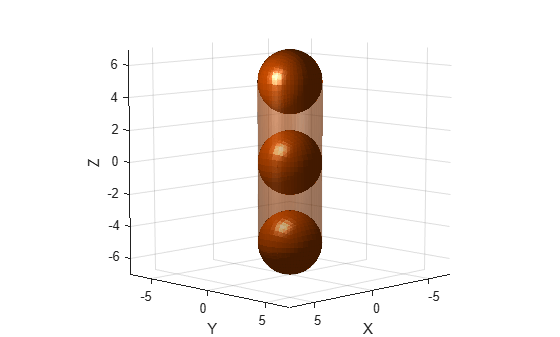# collisionCapsule

Capsule primitive collision geometry

Since R2022b

## Description

The `collisionCapsule` object is a capsule primitive collision geometry defined by a radius and length. The central line segment of the capsule aligns with its z-axis. The origin of the body-fixed frame is at the midpoint of the central line segment of the capsule.

## Creation

### Syntax

``CAPS = collisionCapsule(radius,length)``
``CAPS = collisionCapsule(___,Pose=pose)``

### Description

example

````CAPS = collisionCapsule(radius,length)` creates a capsule primitive with the specified radius `radius` and length `length`. The `radius` and `length` arguments set the `Radius` and `Length` properties, respectively```
````CAPS = collisionCapsule(___,Pose=pose)` sets the `Pose` property of the capsule to `pose`, relative to the world frame.```

## Properties

expand all

Radius of the spherical ends of the capsule, specified as a nonnegative scalar. Units are in meters.

Example: `2.5`

Length of the central line segment of the capsule, specified as a nonnegative scalar. Units are in meters.

Note

This is not the length from end-to-end of the capsule. The total length of the capsule is `Length+2(Radius)`.

Example: `4.5`

Pose of the capsule relative to the world frame, specified as a 4-by-4 homogeneous transformation matrix. Units are in meters.

Example: `trvec2tform([4 2 5])`

## Object Functions

 `checkCollision` Check if two geometries are in collision `convertToCollisionMesh` Convert collision primitive geometry into collision mesh geometry `genspheres` Generate spheres along central line segment of capsule `show` Show collision geometry

## Examples

collapse all

Create a collision capsule with a radius of `2` and length of `10`. Visualize the capsule.

```cCapsule = collisionCapsule(2,10); [~,p] = show(cCapsule);```

Generate spheres at ratios `0.0`, `0.5`, and `1.0` of the capsule length.

`spheres = genspheres(cCapsule,linspace(0,1,3));`

Display the positions of the spheres.

```for i = 1:length(spheres) disp(tform2trvec(spheres{i}.Pose)) end```
``` 0 0 -5 0 0 0 0 0 5 ```

Set the face and edge alphas of the capsule to low values. This ensures that both the spheres are visible when you add them to the figure.

```p.FaceAlpha = 0.4; p.EdgeAlpha = 0.01; hold on```

Display the generated spheres on the capsule.

`cellfun(@show,spheres);`## Version History

Introduced in R2022b

expand all